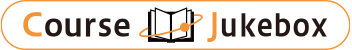### CourseDetailDegree
Master
1, 2
Course delivery methods
face-to-face
Subject
Physical sciences, Mathematical sciences
Program
School
Master's Program in Physics
Department
Master's Program in Physics
Campus
Tsukuba Campus
Classroom
1K118
Course Offering Year
2023-2024
Course Offering Month
October - December
Weekday and Period
Mon/Thu3
Capacity
Credits
2.0
Language
English
Course Number
01BC096

### Computational Physics University of Tsukuba

#### Course Overview

Learn basics of computational particle physics, astrophysics, and biophysics. In computational particle physics, we will start with the basics of Monte Carlo simulations and give an overview of the calculation of hadron mass spectrum by QCD simulation. In computational astrophysics, we will explain the numerical simulation of fluid dynamics, which is important for celestial body formation, from the basics. In computational biophysics, we give an overview of electronic state calculations based on molecular dynamics and density functional theory.

#### Learning Achievement

Learning basics of computational particle physics, astrophysics, and biophysics.

#### Competence

Related to a generic competence "1. knowledge utilization ability" as well as specific competences "1. specialized utilization ability", "2. physical ability" and "4. logical ability".

#### Course prerequisites

Quantum mechanics and statistical mechanics at the undergraduate level are required.

The final evaluation is given based on the average scores of three reports for computational particle physics, astrophysics, and biophysics.

#### Course schedule

In computational particle physics, we will start with the basics of Monte Carlo simulations and give an overview of the calculation of hadron mass spectrum by QCD simulation. In computational astrophysics, we will explain the numerical simulation of fluid dynamics, which is important for celestial body formation, from the basics. In computational biophysics, we give an overview of electronic state calculations based on molecular dynamics and density functional theory.
Computational particle physics: quantum field theory and statistical mechanics
Computational particle physics: basics of Markov chain Monte Carlo methods
Computational particle physics: applications of Markov chain Monte Carlo methods
Computational particle physics: quantum field theory on the lattice
Computational particle physics: formulation of lattice QCD
Computational particle physics: lattice QCD calculations (Physical observables and continuum limit)
Computational astrophysics: basics of fluid dynamics
Computational astrophysics: numerical solutions of linear advection equations (basics)
Computational astrophysics: numerical solutions of linear advection equations (applications)
Computational astrophysics: numerical solutions of fluid equations (basics)
Computational astrophysics: numerical solutions of fluid equations (applications)
Computational astrophysics: numerical simulations of gravitational systems and cosmic plasma with Vlasov equation
Computational biophysics: molecular dynamics
Computational biophysics: an overview of electronic state calculations in many-body systems
Computational biophysics: density functional theory
Computational biophysics: Kohn-Sham equation
Computational biophysics: exchange-correlation functionals
Computational biophysics: electronic state simulations for atoms
Exercise
Exercise

Lectures

#### Instructor

Ohno Hiroshi,Shoji Mitsuo,Yajima Hidenobu

#### Other information

English lectures on request.